## Introduction

Nuclear magnetic resonance (NMR) quantum computing has attracted broad interest because it is one of the most advanced testbeds for quantum computation. Although the interest began with solution NMR1,2,3, it is now believed that scalable NMR quantum computers in the future will be built on semiconductors based on highly developed semiconductor technology4,5,6. The main challenges include the initialization and the creation of spin entanglement, which are essential features of quantum computation7. Semiconductor-based NMR quantum computers are advantageous as they can be achieved optically; that is, the initialization (nuclear–spin polarization) is provided by optical pumping8,9, and the entanglement is created via internuclear (nuclear spin–spin) couplings between polarized nuclei10,11. In optically pumped semiconductors, the latter manifests itself as dipolar order12,13 and double-quantum coherence14.

Switchability is another essential functionality required for internuclear couplings, which should be 'on' during operations and 'off' otherwise. In this respect, nuclear dipolar coupling (D-coupling, hereafter) is not the best choice for the above-mentioned reasons. In addition, the time required for operations increases rapidly with increasing qubit number because of decoupling operations15. Other possible candidates include indirect couplings mediated by donor electrons4 and magnons15. Their implementations are fairly challenging, however, given the complicated switching mechanisms. By contrast, the scheme presented in this paper is rather simple; the coupling strength can be controlled externally through light power, and on/off switching can be easily implemented.

In this study, we have preformed cross-polarization (CP) experiments with GaAs under light illumination, and demonstrated that a nuclear spin–spin coupling grows in strength, and extends its reach to farther nuclei as light power is increased. These futures bring about a unique transition of the CP process from oscillatory behaviour in the 'dark' towards exponential relaxation with increasing light power. The experiments provide us with information on the essential features of the optically induced nuclear spin–spin couplings; in particular, we find that the coupling strength is roughly proportional to light power, which is essential for the switching of the couplings.

## Results

### CP process in GaAs in the dark

The present mechanism is manifested in a CP process from 75As (I-spin) to 71Ga (S-spin) in GaAs under infrared light irradiation. Before detailing this process, we first describe it in the dark (without light irradiation) as a reference. This is an ordinary CP process, for which we expect a contact time (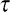cp) dependence of S-magnetization (MSeq) of the formwhere TIS is the cross-relaxation time. A relaxation process in the rotation frame (T) need not be considered here, as it is sufficiently long because of high crystal symmetry16,17. The reality, however, is more complicated than equation (1). Figure 1 shows MSeq(cp) obtained in the dark, which exhibits a clear transient oscillation.

Transient oscillations have been reported in some molecular crystals, and attributed to discrete S–I coupling spectra of isolated S–I pairs18,19,20. The magnetization is transferred back and forth inside the pair with a frequency corresponding to half the flip-flop term of the D-coupling. The present sample, however, is not a molecular crystal, so isolated pairs are expected to be rare. Here an essential factor is the existence of two Ga isotopes, that is, 69Ga and 71Ga with natural abundances of 69NA=0.604 and 71NA=0.396, respectively. A local 75As -71Ga pair appears when only one of the four nearest-neighbour sites of 75As is occupied by 71Ga and the others are occupied by 69Ga. The probability of finding such pairs is 4C1·71NA·(69NA)3=0.35; that is, about 35% of 75As have a single 71Ga in the vicinity and contribute to the oscillation. The pairs are coupled through indirect scalar coupling JIS, where D-couplings are absent because the Ga sites are situated at magic angle positions in the (100) crystal orientation16,17,21. The process is described by a damping oscillation18,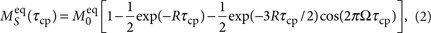where Ω is the oscillation frequency (Ω=JIS/2) and R is the damping factor. We fit the data using equation (2) with Ω and R as free parameters. The best result is obtained with Ω=0.74 kHz, which yields JIS=2Ω=1.48 kHz. This value is comparable with that in the InP case (JIS=2.3 kHz)17. The fitting curve is shown by a solid line in Figure 1. The fit is not very good at the beginning of the oscillation; this may be due to the presence of 75As sites with more than one 71Ga nucleus in the four nearest-neighbour sites.

### CP process under light irradiation

Figure 2a shows thecp dependence of S-polarization, MS(cp) under various levels of light power, PIR, which exhibits new local maxima (peaks) that are not observed in the dark. The maxima form a number of series (α, β, γ...), and the maximal position in each series shifts towards smaller values ofcp as the light power is increased. For example, the series 'β' shown by the red arrows starts with a broad maximum aroundcp=3.1 ms at PIR=100 mW, which shifts towards smaller values ofcp as PIR is increased and eventually merges into a peak atcp=0.8 ms at 166 mW. These maxima represent new polarization transfer processes that appear under light irradiation. Figure 3 shows a three-dimensional representation of Figure 2a obtained by interpolating the data in between. The continuous shift of the maximum in each series can be readily confirmed.Figure 3: Light power dependence of the CP process in GaAs.

This phenomenon can be explained by discrete increments in the number of S-nuclei (71Ga) involved: in the dark (PIR=0 mW), the number of nuclei participating in the process is small and oscillatory behaviour is observed, as shown in Figure 1. Light illumination causes a series of S-nuclei batches (α, β, γ...) to participate in sequence. Their contributions are successively added to the signal intensity one after another, whereas the speed of transfer from I- to S-nuclei in each series increases with the light power (that is, the maximal position shifts towards smaller values ofcp). As the number of nuclei involved is further increased, the cross relaxation is expected to approach the exponential relaxation behaviour given by equation (1)20. That is, we see here the transition from oscillatory behaviour in the 'dark' towards exponential relaxation with increasing light power. This is a unique case in that such a transition can hardly be observed in ordinary NMR measurements.

Another factor responsible for the phenomenon is the optical pumping effect8,9. It contributes to the S-magnetization (71Ga) through the enhancement of the I-magnetization (75As) caused by the polarization transfer from the optically oriented electrons for the duration of light irradiationL=60 s. Figure 2b shows the light-power dependence of the I-magnetization (75As), which increases linearly with light power. This enhancement is partly responsible for the increase of the S-magnetization with increasing light power, as seen in Figures 2a and 3.

Thecp dependence of Ms is expressed as,where MIdark(L) in the first term on the right-hand side represents the I-magnetization in the dark portion of the sample recovered duringL=60 s and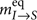represents the polarization transfer process to S-spins in the first nearest neighbour sites through JIS. This process is essentially the same as that in Figure 1. On the other hand,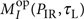in the second term is the I-magnetization in the illuminated area generated by the optical pumping effect duringL=60 s, whose PIR dependence is shown in Figure 2b. This magnetization is transferred to S-spins through the two processes shown in the brackets: the same process as that in the dark,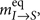, and additional ones induced by light irradiation,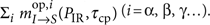. The latter are caused by the optically induced heteronuclear indirect coupling,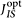. We will discuss characteristics of this coupling in the next section.

## Discussion

The new couplingis presumably mediated by photo-excited electrons. It is known that electrons in metals can mediate indirect nuclear spin–spin couplings, a process referred to as the RKKY interaction22. In the present case, however,is observed in semiconductors where no intrinsic Fermi surfaces exist. Moreover, the lifetimes and spin relaxation times of the photo-excited electrons usually fall in the range between pico- and nanoseconds, which is more than six orders of magnitude smaller than that of the CP process, that is, milliseconds. It is intriguing that two phenomena with such different time scales are coupled with each other.

The strength ofin each batch (α, β, γ...) can be evaluated by the cross-relaxation time TISopt in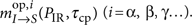. According to Demco et al.17,20,23, the cross-relaxation time is expressed aswherec is the correlation time of the CP and,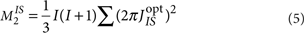is the second moment of the I–S heteronuclear spectrum due to. Equation (5) indicates that M2IS is proportional to ()2. Provided thatis proportional to PIR and thatc is independent of, equations (4) and (5) lead to the conclusion that 1/TISopt is proportional to PIR2. The actual value of TISopt in each batch is determined from the analysis of the functional form of. Here we evaluate it from the maximal position; TIS* is defined as the contact time at which the maximum is formed. Figure 2c shows 1/TIS* for the three series of maxima (α, β and γ) plotted against PIR2. The graph suggests that 1/TIS* is roughly proportional to PIR2, implying thatis proportional to PIR.

This result provides a clue to the nature of the series of S-nuclei batches (α, β, γ...). Equation (5) indicates that the condition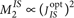is fulfilled only when all I–S pairs participating in the summation of the right-hand side share the same, such thatcan be taken out of the summation Σ. Therefore, each series may be assigned to the polarization transfers to a batch of S-spins at the same distance from the I-spin at the origin. Figure 3 illustrates this situation. For example, the α-maxima may be assigned to the second nearest-neighbour S-spins, the β-maxima to the third, and so forth. It is reasonable that equivalent S-spins in the same order situated at the same distance from the I-spin share the same scalar coupling with it. Moreover, the contributions from nuclei in the same order to the correlation time are partially cancelled out17,20,23. Therefore, the assumption thatc is independent ofmay be a reasonable approximation.

The result also provides us with information about the reach of. The dotted red line in Figure 3 shows the PIR dependence of Ms(cp) at a constantcp. One finds a plateau-like feature around 80 mW, which indicates that the S-spins at the third nearest-neighbour sites participate in the process only when PIR>80 mW; that is,can reach farther than D- and JIS-couplings, as the latter two may not substantially reach the third-nearest neighbours.

Features such as the external switchability and the long reach may add variety to the qubit arrangement in semiconductor-based NMR quantum computers. Figure 4 shows an example. In an array of nuclear–spin qubits, a few lattice points apart from each other, the D-couplings are out of reach, so that the qubits are decoupled. Then, the present mechanism provides the external control of internuclear couplings. As the light power is increased, the reach of couplings is extended. This would further enhance the flexibility of qubit arrangements.

This mechanism is compatible with most schemes proposed for semiconductor-based NMR quantum computing. The implantation of nuclear spin arrays in semiconductors has been studied using various techniques such as scanning probe microscopes, ion beams, and isotope engineerings24,25,26. These techniques will provide promising technologies to implement the schemes shown in Figure 4.

In conclusion, we have discovered an optically switchable indirect nuclear spin–spin coupling, which is manifested in CP experiments with GaAs under light illumination. As the strength of this coupling is externally controllable through light power, we expect it to have an essential role in the quantum gate operations of solid-state NMR quantum computers in the future.

## Methods

### Optical pumping double resonance NMR system and the sample

The CP experiments were performed at 10 K using an optical-pumping double-resonance NMR system developed for this study. The system consists of an NMR spectrometer, a laser system and a cryostat loaded in a 6.34-T superconducting magnet. A custom-built double-resonance probe is installed inside the cryostat. The sample used in this study is an undoped semi-insulating GaAs single-crystal wafer with a thickness of 600 μm and a crystal orientation of (100) (Mitsubishi Chemical). It is mounted on a sample stage made of sapphire located at the probe head and set with the surface normal to the magnetic field. The sample stage is cooled through thermal contact with the cold head of the cryostat. Infrared light emitted from a Ti:Sapphire laser is delivered to the cryostat through a polarization-maintaining fibre27. It is converted to circularly polarized light by a quarter-wave plate at the probe head, and applied to the sample in parallel to the magnetic field. The spot size at the sample surface is about φ5 mm.

### Pulse sequence

The pulse sequence used is shown in Figure 5. The magnetization of I-spins, saturated by the first comb pulse, is regenerated during the time intervalL and transferred to S-spins through the CP immediately thereafter. The infrared light is irradiated at a constant strength PIR throughout the sequence. The photon energy of 1.50 eV (near the band gap) and the helicity σ were selected so that the optical pumping NMR signal enhancements for both 71Ga and 75As were nearly maximal. The experiment in the dark (Fig. 1) was obtained with the same pulse sequence as that in Figure 5, with the exception that PIR=0 mW.